# Department of Gravimetry and Geodynamics

Web page of the Department of Earth Science Institute SAS

## The basic tasks of geothermal research

Written by  Dušan Majcin | Published in: Geothermics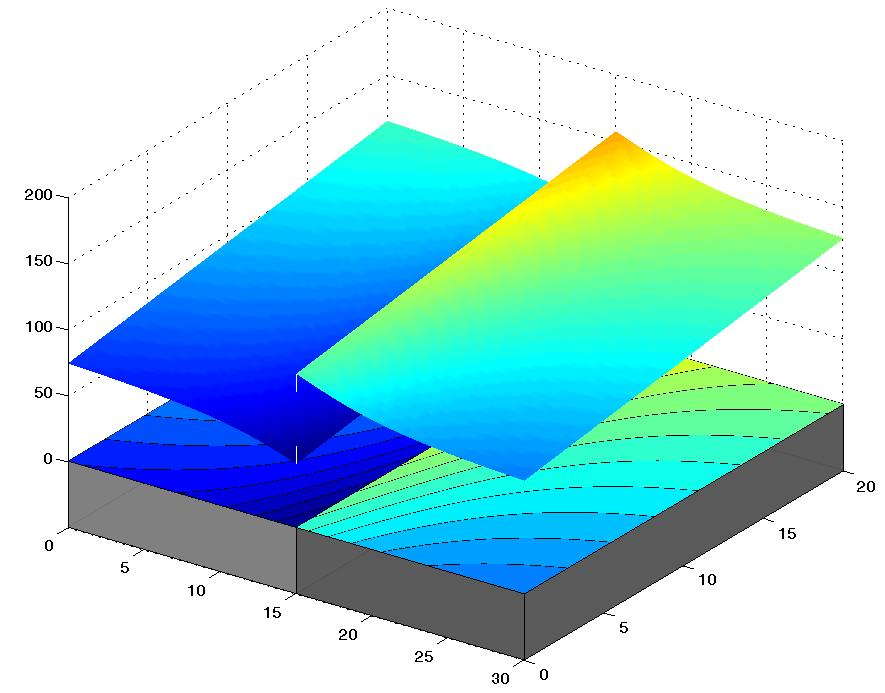The solution of the problems of basic research of the temperature distribution and of the heat flow density components distribution caused by various types of the refractions and/or of the heat sources. The math-physical tasks in bounded 2D/3D areas are solved by means of finite difference, by finite element approaches, and by boundary elements methods.  The temperature fields modeling results are utilized by interpretation of the data describing the thermal state of the lithosphere in the region of the Western Carpathians. The interpretation approaches are adapted both to concrete structural and tectonic conditions and to available geological and geophysical data.

Presented are some selected examples of the activities within the basic geothermic research.

1.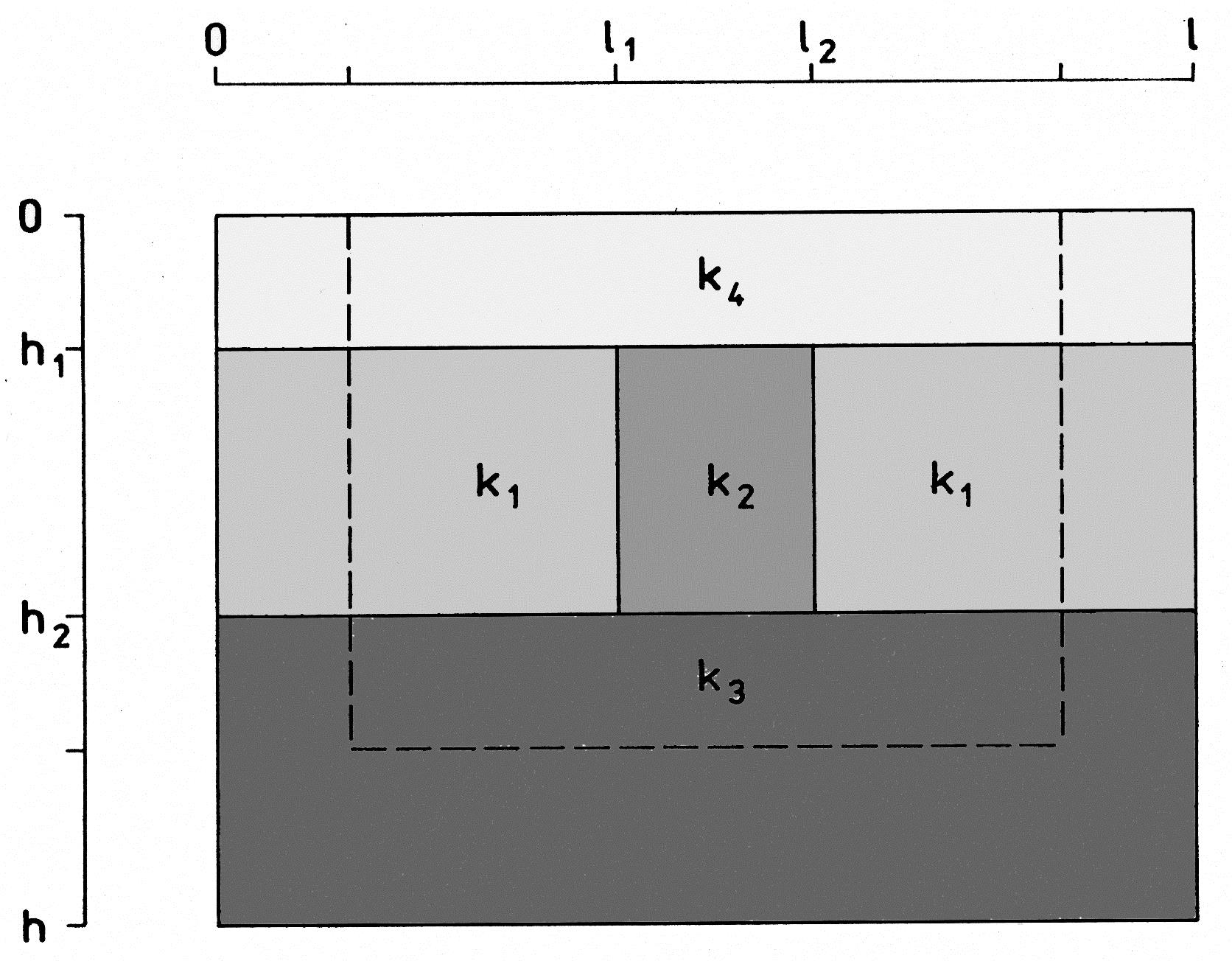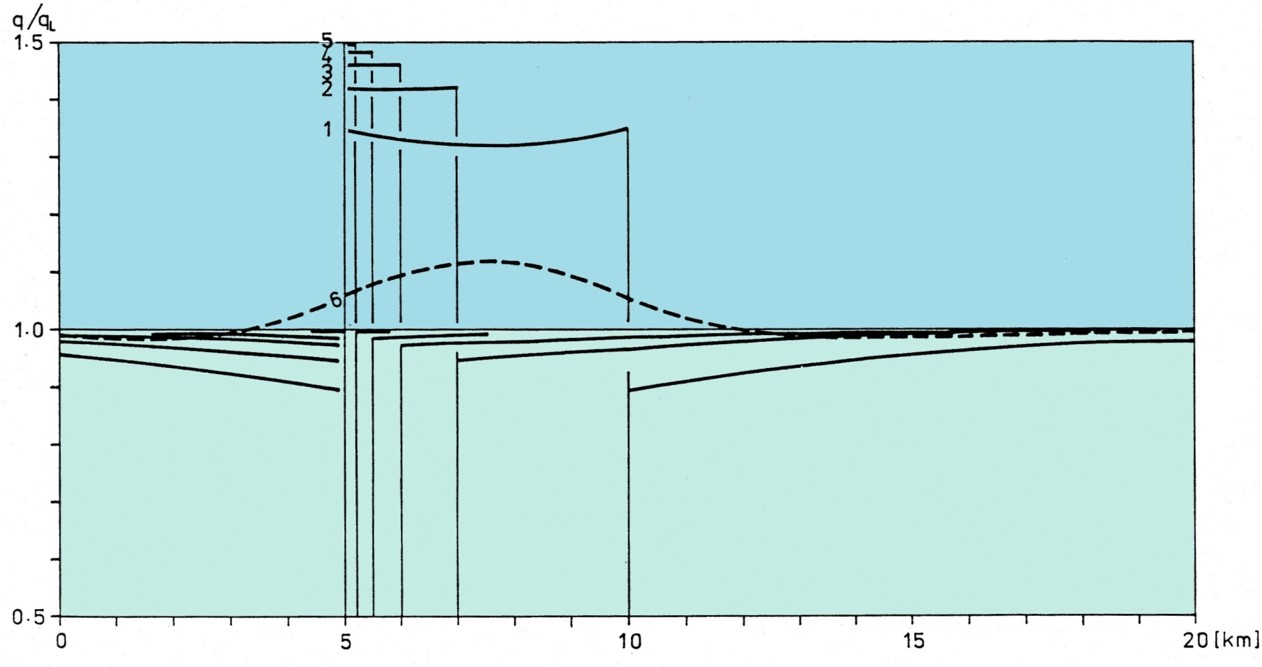Basic problems of the heat flow refraction on simple structures with various combinations of the thermal conductivity parameters.

Displayed are the relative surface heat flow density distributions for models of vertical structure (upper picture) with various depth b = l₂ - l₁,with covering layer (h₁ = 2000 m, thick dashed line in lower picture) and for comparison also without it (h₁ = 0 m, solid lines). In shown models there are fixed some thermal conductivity data and space dimensions of model k₂ / k₁ = 3.00/2.00, h₂ = 5000 m, k₂ = k₃.  The width b ischanged for separate models:for 1 and 6 b = 5000; for 2 b = 2000; for 3 b = 1000; for 4 b = 1000; for 5 b = 500 m. The distributions of the surface heat flow density for models without covering layer contain the step above the border of anomalous body. The existence of covering layer caused only local anomaly without the step change. The step in the distribution responds to change in thermal conductivity values. The curvature of distribution graphs is caused mainly by changes in temperature field distributions for corresponding models. The narrow body the smaller changes exist in the temperature field. This has a great importance by interpretation of the geothermal characteristics (heat flow density and temperatures) in surroundings of fault zones.

2.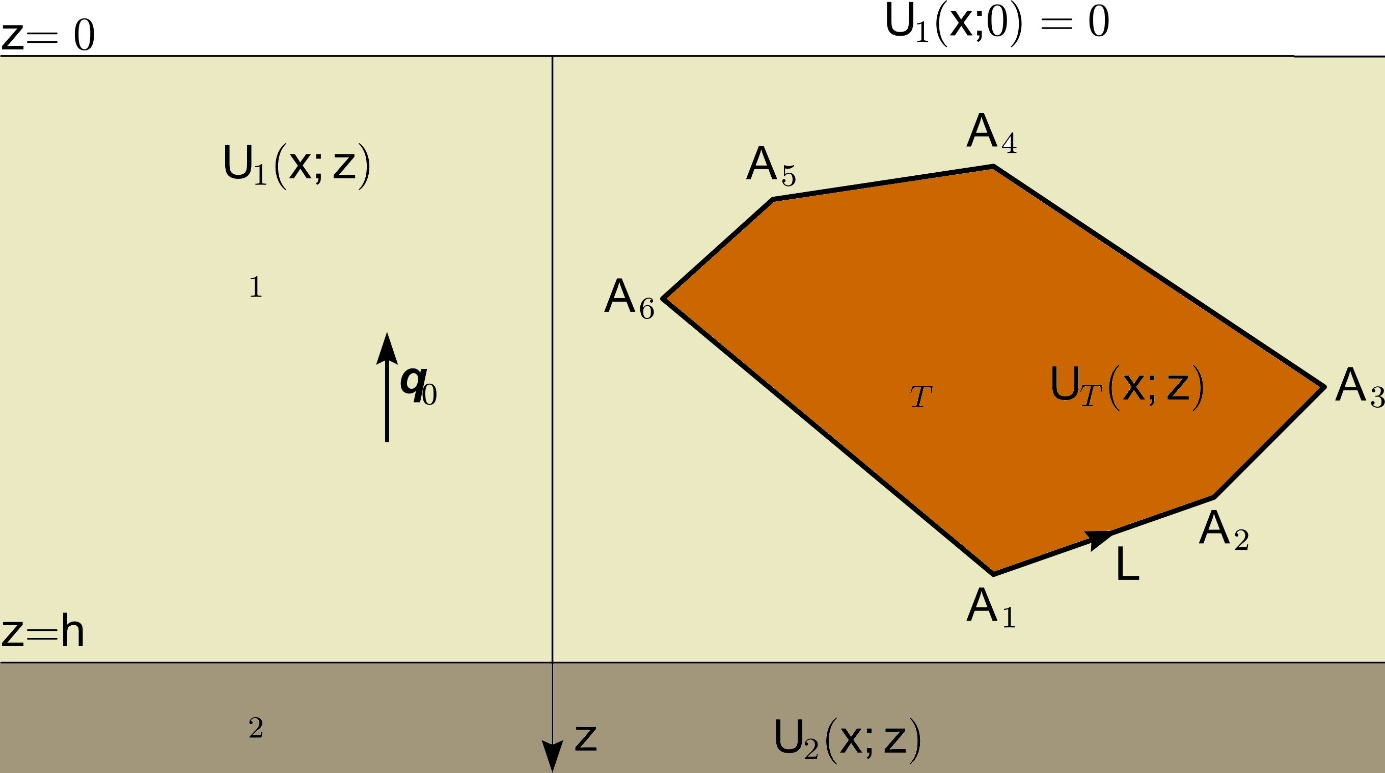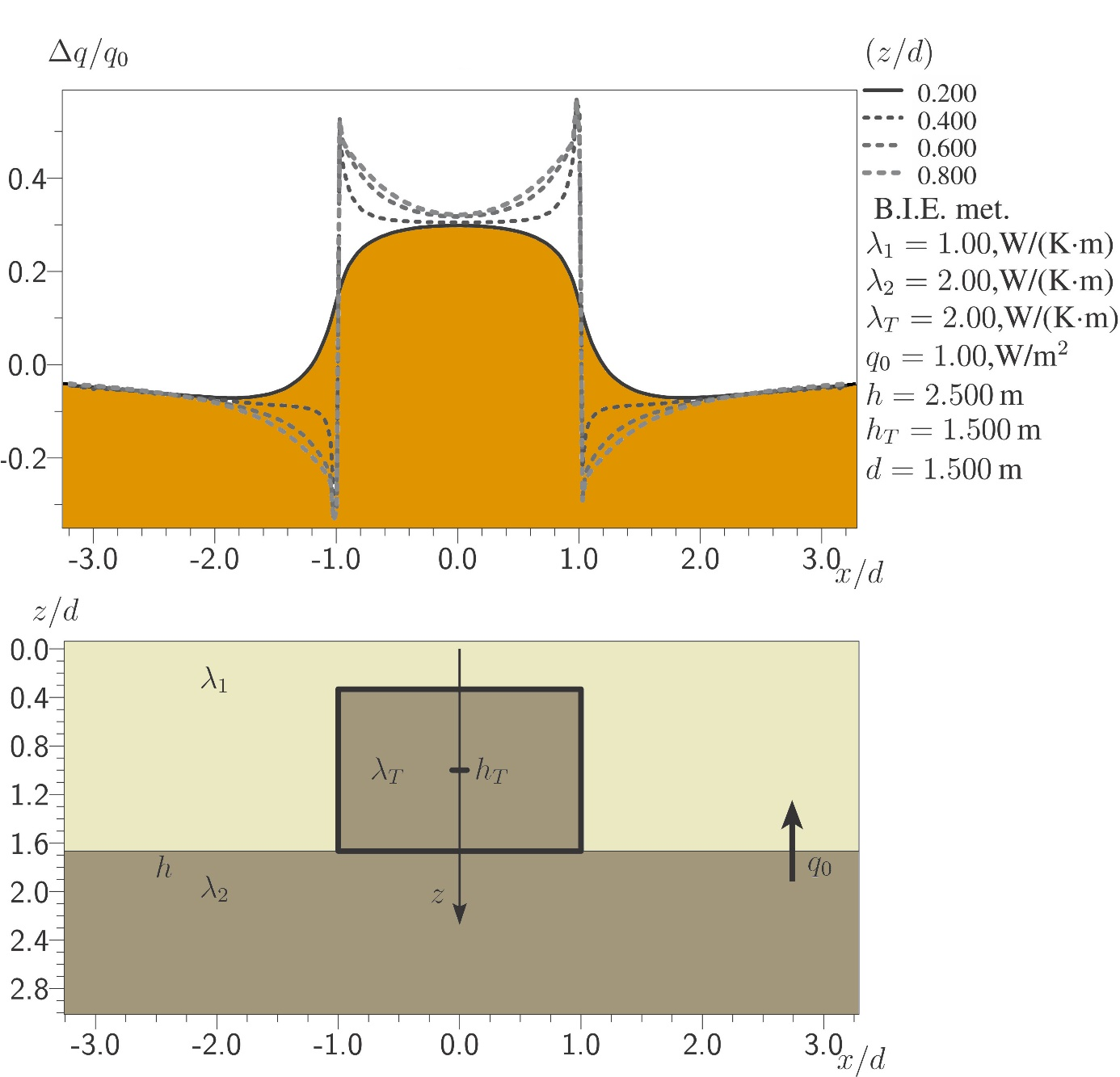Distributions both of the relative surface heat flow density and of the relative heat flow density on various horizontal levels for refraction on the 2D polygonal body in two-layer Earth (upper picture).

Sample variant of the model body with contact on the boundary of layers (approximating the horst structure with vertical lateral boundaries). Model configuration and results are shown in two lower pictures. The step change in distributions exist only in horizontal levels crossing the horst. Such models are utilized for interpretations of temperature distributions and calculated heat flow density values in boreholes lying closely to bodies with different thermal conductivity coefficient.

3.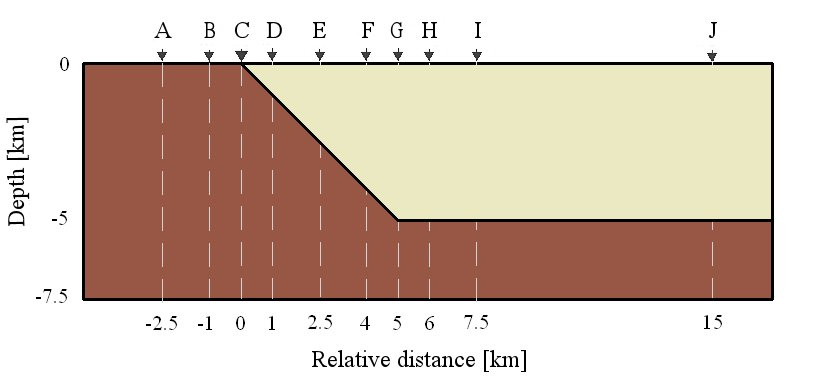Depth distributions of the relative vertical heat flow density component in check points A - J due to heat flow refraction for basic basin margin model (upper picture). Individual presented curves respond to distributions of calculated heat flow densities (with step of 100 m) in boreholes coming through the structure (lower picture). The step change is typical for aslant boundaries between rock complexes and moreover influence of the horizontal heat transport on structure is noticeable.

The result has a great importance by the determination of heat flow data near the basin filling / basement boundary. The estimation of the refraction effects is utilized for interpretations of terrestrial heat flow density distributions near the sedimentary basins borders i.e. by successive stripping inversion methodology in the geothermal modeling.

4.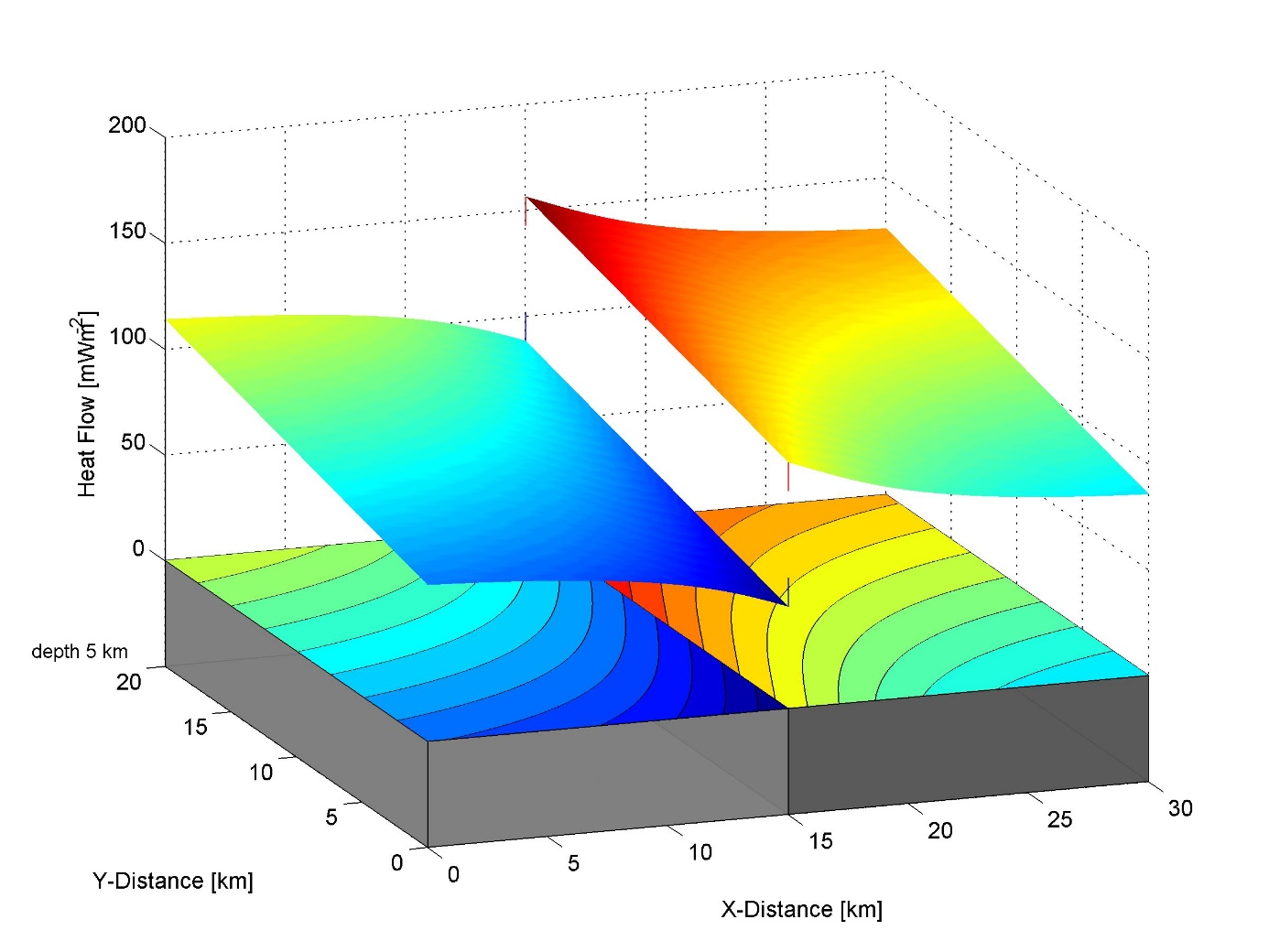Step change in distribution of the heat flow density vertical component caused by flow refraction on the vertical interface boundary with thermal conductivity coefficient contrast and by supposed linear increase of heat flow density values at the lower model boundary along the interface plane with contrast.  The directions of 2D isolines on both sides of interface are the same (this responds to continuous temperature distribution) however the shift of isolines of the same value exists over the interface line (this is caused by step change of the thermal conductivity at interface plane of rock complexes). The thermal conductivity of rock complexes in presented model is defined as follows:  light gray - 2 W/m.K and dark gray - 3 W/m.K.

5.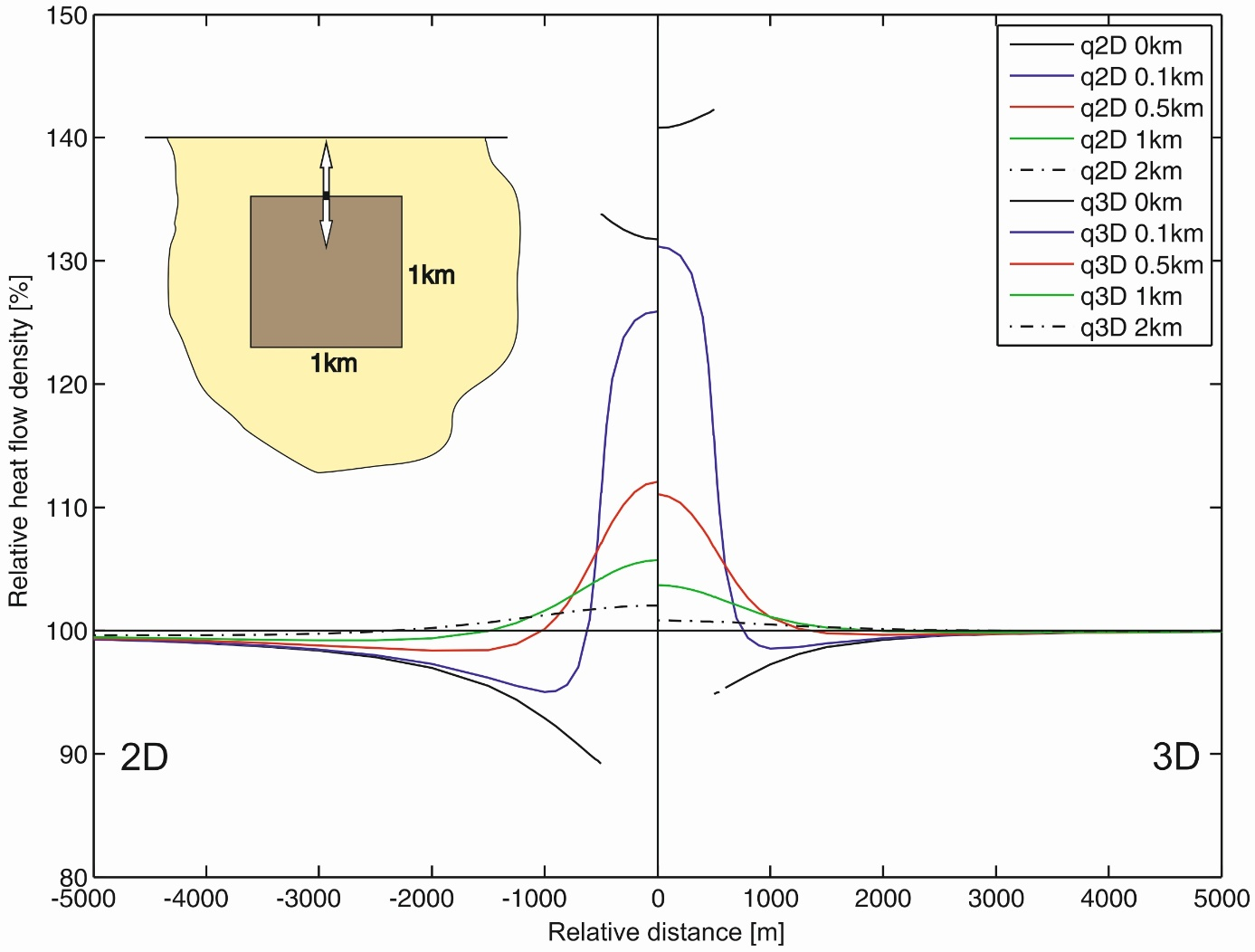The comparison of the relative surface heat flow distributions for refraction on 2D and 3D anomalous body placed in the half-space at various depths (listed in figure legend) of upper body face. Left side: 2D model with anomalous parallelepiped of rectangular cross section. Right side: 3D model with anomalous cube. The calculation results provide interesting specious paradox in surface heat flow density distributions – the anomaly amplitude is greater over smaller 3D body than that one over 2D body always when the anomalous body is situated on or near the surface of the halfspace. This is a specific property of refraction anomalies caused by the fact that 3D body concentrates the streamlines from more directions.  In models with greater depth positions of bodies there the amplitudes of heat flow anomalies for 2D and 3D bodies behave similar as in source problems.

6.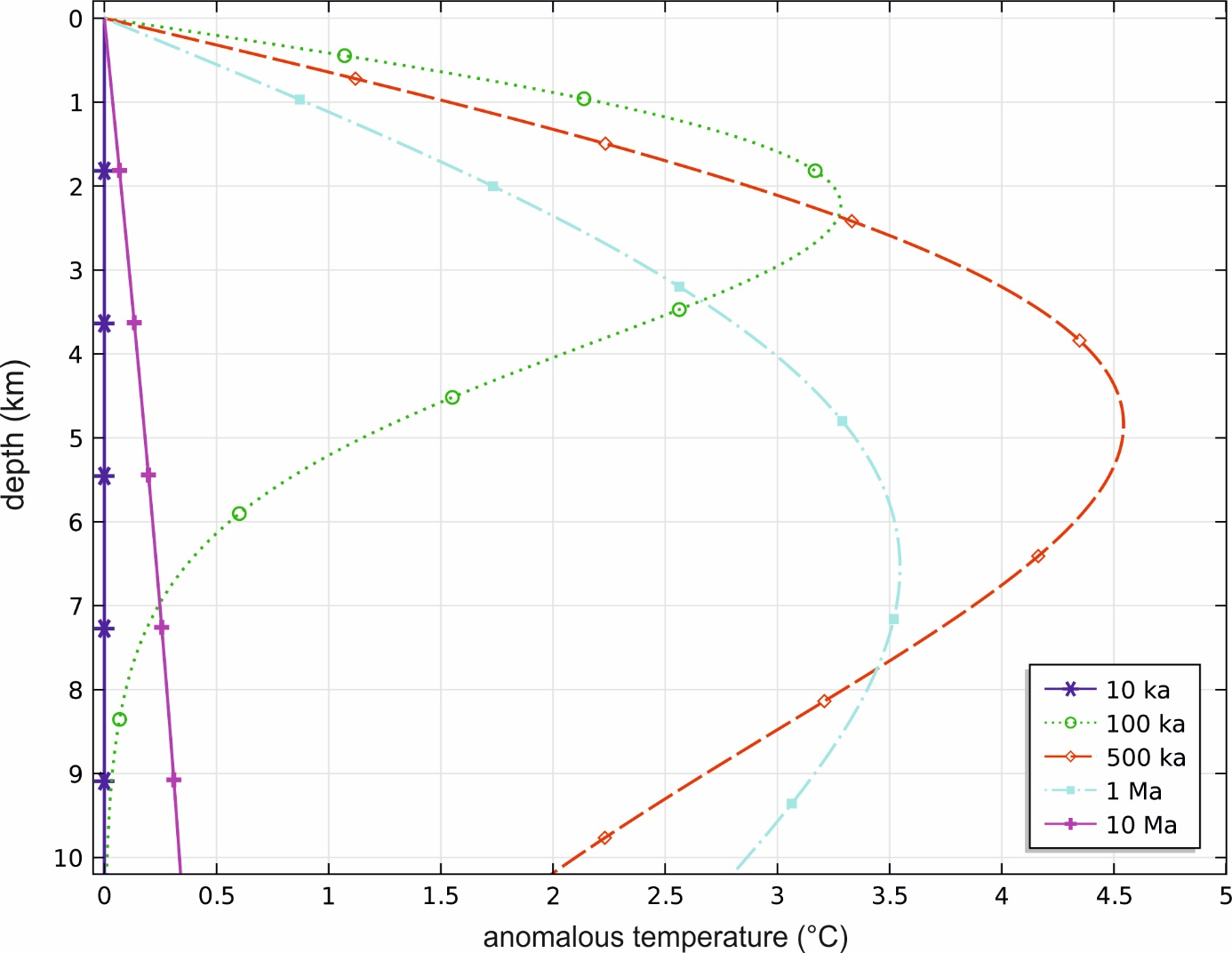Time changes in anomalous temperature depth distribution for cooling of subvolcanic body situated in the depth 1 km. The thickness of the sill part of the body is about 1 km.

Such estimation of magmatic bodies cooling effects are used by successive stripping inversion approaches in the geothermal modelling together with estimations of refraction effects.

## References

Majcin D., 1982: Mathematical models of stationary heat conduction. Contrib. Geophys. Geod., 13, 135-151.

Majcin D., 1993: Refraction of heat flow on the near-surface structures with thermal conductivity contrast. Contributions - Slovak Academy of Sciences, Geophysical Institute 22, 67-80.

Majcin D., Polák Sz. 1995: Refraction of heat flow near the border of the sedimentary basins with topography. Contrib. Geophys. Geod., 25, 99-112.

Tsvyashchenko V. A., Majcin D., Kutas R. I., Tsvyashchenko A. V., 2000: Modelling stationary thermal field of heterogeneous environment. Doklady NAS of the Ukraine, 4, 134–138 (in Russian).

Hvoždara M., Majcin D., 2009: Geothermal refraction problem for 2-D body of polygonal cross-section buried in the two-layered earth. Contributions to Geophysics and Geodesy, vol. 39/4, 301-323.

Hvoždara, M., Majcin D., 2011: Refraction effect in geothermal heat flow due to a 3D prismoid, situated in the substratum of two-layer Earth. Contributions to Geophysics and Geodesy, vol. 41/3, 211-233.

Majcin D., Bilčík D., Hvoždara M., 2012: Refraction of heat on subsurface contrast structures – the influence both on geothermal measurements and on interpretation approaches. Contributions to Geophysics and Geodesy, vol. 42/2, 133-159.

Hvoždara M., Majcin D., 2013: Geothermal heat ﬂux anomaly due to a 3D prismoid situated in the second layer of a three-layered Earth. Contributions to Geophysics and Geodesy, vol. 43/1, 39-58.

Majcin D., Bilčík D., Kutas R.I., Hlavňová P., Bezák V., Kucharič Ľ., 2014: Regional and local  phenomena inﬂuencing the thermal state in  the Flysch belt of the northeastern part of Slovakia. Contrib. Geophys.  Geod., 44/4, 271–292.